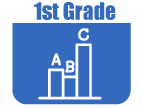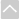Review Activities and Games

These resources are intended for review and practice after initial instruction of the standards.

Strand: MATHEMATICAL PRACTICES (1.MP)Strand: OPERATIONS AND ALGEBRAIC THINKING (1.OA)
Represent and solve problems involving addition and subtraction within 20 (Standards 1–2, 5–6).
• \$20 Dot Map
This problem helps students to practice adding three numbers whose sum are 20 or less. It is an open-ended problem with many solutions. This problem would work well either as a whole group or students could work on it as individuals or pairs.
• At the Park
This task includes three different problem types using the "Add To" context with a discrete quantity.
• Boys and Girls, Variation 1
This task represents the Put Together/Take Apart contexts for addition and subtraction. Students may use either addition or subtraction to solve these types of word problems, with addition related to the action of putting together and subtraction related to the action of taking apart.
• Boys and Girls, Variation 2
• Finding a Chair
This task represents compare contexts for addition and subtraction. The problem explicitly describes one-to-one correspondences without using comparison language.
• Growing Bean Plants
This task adds some rigor to this common activity, by collecting actual growth data, providing practice for students in measuring and recording length measurements.
• IXL Game: Addition: Word problems
This game helps first graders understand addition using word problems. This is just one of many online games that supports the Utah Math core. Note: The IXL site requires subscription for unlimited use.
The purpose of this task is for students to identify and represent related addition and subtraction equations with objects and equations.
• Making a ten
This task is designed to help students visualize where the 10's are on a single digit addition table and explain why this is so. This knowledge can then be used to help them learn the addition table.
• Maria's Marbles
This task includes problem types that represent the Compare contexts for addition and subtraction. The student must solve word problems involving marbles.
• Measuring Blocks
This task has students work in pairs. Given two or more blocks of different lengths and paper clips to use to measure them, the pair measures the blocks and tell how many paper clips long it is. They then figure out how long two different blocks would be when laid end to end and explain how they solved the problem.
• Peyton's Books
This task was designed to help students to make sense of problems and persevere to solve them, as well as understanding the relationship between addition and subtraction. Students will solve the take from, change unknown problem, and through a teacher-facilitated discussion, understand that the problem can be solved using addition or subtraction.
• School Supplies
In solving a word problem about school supplies, this task could be used for either instructional or assessment purposes, depending on where students are in their understanding of addition.
Understand and apply properties of operations and the relationship between addition and subtraction (Standards 1.OA.3–4).
• Cave Game Subtraction
The purpose of this task is for students to practice creating and memorizing subtraction equations, while focusing on missing addends.
The purpose of this task is to help students understand the commutative property of addition. Because the total number of dots is the same regardless of how a domino is oriented, the domino reinforces the idea that the addends can be written in any order.
• Fact Families
The purpose of this task is for students to identify and write sets of related addition and subtraction equations; these are often known as "fact families" because the equations are related by the same underlying relationship between the numbers. This task reinforces the commutative property of addition and using the relationship between addition and subtraction.
• Fact Families with Pictures
The purpose of this task is for students to reinforce students' understanding of "fact families." Fact families reinforce the commutative property of addition and using the relationship between addition and subtraction. Working with fact families is a common activity, although often times students are asked only to work with symbols. Also, the scaffolding for these tasks often only supports students in writing four of the eight possible facts in a family; this task purposefully scaffolds them to write all eight. In addition, this task includes a picture to anchor each fact-family; students can graduate from here to a symbols-only version of this task
Represent and solve problems involving addition and subtraction within 20 (Standards 1–2, 5–6).
• \$20 Dot Map
This problem helps students to practice adding three numbers whose sum are 20 or less. It is an open-ended problem with many solutions. This problem would work well either as a whole group or students could work on it as individuals or pairs.
• Making a ten
This task is designed to help students visualize where the 10's are on a single digit addition table and explain why this is so. This knowledge can then be used to help them learn the addition table.
Work with addition and subtraction equations (Standards 1.OA.7–8).Strand: NUMBER AND OPERATIONS IN BASE TEN (1.NBT)
Extend the counting sequence (Standard 1.NBT.1).
This game is a version of the traditional memory or concentration game. Students will us a set of number cards for each of the pair of numbers that cross the decade, i.e., 19 and 20, 29 and 30, 39 and 40, 49 and 50, etc. Students place all the number cards that end with 9 face down in an 3x3 array on the left and all the number cards that end with 0 face down in a 3x3 array on the right. Working in pairs or trios, students take turns. The first student selects a card from the left array, stating the number name and the counting number that follows ("I have 39, I need 40"). When a student finds a matching pair he or she keeps that pair of cards. Play continues until all cards have been matched. The student with the most matched pairs wins.
• Choral Counting II
In this activity students work with a large number line and chant a counting sequence when led by the teacher using a pointer.
• Hundred Chart Digit Game
This task is a bingo-like card game where students draw numbers and then mark the spaces on their card for both numbers that can be made with those digits.
• Number of the Day
This activity provides a connection between the counting sequence and an experience from students' daily lives. It helps to give the students a sense of how "many" each number is.
• Start/Stop Counting II
This activity has the teacher walking around the room while counting aloud from a number between 1 and 20. The teacher continues to count until he/she chooses a student by patting them on the shoulder. The student and teacher switch roles, the teacher sits in the studentâs chair while the student resumes the count and walks around the room. At the teacherâs signal the student selects the nearest student and switches places with another child who continues the count. Repeat this until each child has had a turn counting.
• Where Do I Go?
This activity involves a card game and is designed to be a short, repeatable activity to build student flexibility with the number sequence.
Understand place value (Standards 1.NBT.2–3).
• Comparing Numbers
This task is a fun partner game in which students practice making two-digit numbers, decide which is greater, and write the appropriate comparison symbolically.
• IXL Game: Counting Tens and Ones
This game helps the first grader understanding counting tens and ones up to 99. This is just one of many online games that supports the Utah Math core. Note: The IXL site requires subscription for unlimited use.
• Roll & Build
The purpose of this task is to give students practice representing two digit numbers with concrete objects to reinforce the meaning of the tens digit and the ones digit. This task works best in partners, however it can played individually.
Use place value understanding and properties of operations to add and subtract (Standards 1.NBT.4–6).Strand: MEASUREMENT AND DATA (1.MD.)
Measure lengths indirectly and by iterating length units (Standards 1.MD.1–2).
• Growing Bean Plants
This task adds some rigor to this common activity, by collecting actual growth data, providing practice for students in measuring and recording length measurements.
• Measure It! Paperclips
This PDF contains 20 images that students can measure with paper clips helping them understand how to measure the length of an object.
• Measuring Blocks
This task has students work in pairs. Given two or more blocks of different lengths and paper clips to use to measure them, the pair measures the blocks and tell how many paper clips long it is. They then figure out how long two different blocks would be when laid end to end and explain how they solved the problem.
Tell and write time (Standard 1.MD.3).
• Clockwise
Practice telling time with this kid friendly interactive clock.
• IXL Game: Match Analog and Digital Clocks
This game helps first graders tell and write time in hours and half-hours using analog and digital clocks. This is just one of many online games that supports the Utah Math core. Note: The IXL site requires subscription for unlimited use.
Represent and interpret data (Standard 1.MD.4).
• Bar Graph Sorter
The objectives of this lesson are to introduce bargraphs and ask students to sort them by a particular attribute.
• Histogram
This activity will help students understand histograms by allowing them to make their own.
Identify the value of coins (Standard 1.MD.5).Strand: GEOMETRY (1.G.)
Reason with shapes and their attributes (Standards 1.G.1–3).
• IXL Game: Same Shape
This IXL game helps first graders distinguish between defining attributes (e.g., triangles are closed and three-sided) versus non-defining attributes (e.g., color, orientation, overall size). This is just one of many online games that supports the Utah Math core. Note: The IXL site requires subscription for unlimited use.The Online Core Resource pages are a collaborative project between the Utah State Board of Education and the Utah Education Network. If you would like to recommend a high quality resource, contact Trish French (Elementary) or Lindsey Henderson (Secondary). If you find inaccuracies or broken links contact resources@uen.org.